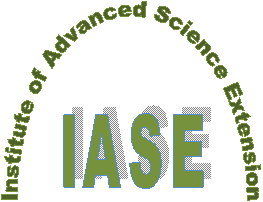International Journal of

EISSN: 2313-3724, Print ISSN: 2313-626X

Frequency: 12Announcement

Call for Papers
Special Issue Announcement: Call for Papers

Trend Topics in Mathematics with Their Applications

Mathematics is the study of topics such as numbers, structure, space, etc. A traditional division of mathematics is into pure mathematics, (mathematics studied for its intrinsic interest), and applied mathematics, (mathematics which can be directly applied to real-world problems). This division is not always clear and many subjects have been developed as pure mathematics to find unexpected applications later on. Broad divisions, such as discrete mathematics and computational mathematics, have emerged more recently. There is a range of views among mathematicians and philosophers as to the exact scope and definition of mathematics. Mathematicians resolve the truth or falsity of conjectures by mathematical proof. When mathematical structures are good models of real phenomena, then mathematical reasoning can provide insight or predictions about nature. Through the use of abstraction and logic, mathematics developed from counting, calculation, measurement, and the systematic study of the shapes and motions of physical objects. The fundamental aim of this special issue is to survey the developments in pure and applied mathematics including analysis, algebra, topology, number theory, approximation theory, dynamical systems, etc. since these topics are studied intensively (by many scientists) over the world. Because of their fruitful applications, we believe that the results derived here will be richly utilized in computational models of scientific, engineering problems, etc. The selected papers in this special issue will be devoted to one of the current trends in mathematics including pure and applied mathematics, mathematical physics, especially will be focused on a wide range of their applications.

Potential topics include:

• Pure and Applied Mathematics (algebra, topology, number theory, approximation theory, mathematical methods in operational research, theoretical statistics and econometrics, and theoretical computer science)

• Mathematical Physics (methods, partial differential equations, dynamical systems, mathematical and numerical aspects of inverse problems)

Nov-30 2019

Serkan Araci, Hasan Kalyoncu University, Turkey

Authors can submit their manuscripts to this special issue via e-mail mentioning the name of this special issue.

Recent Developments in Special (or q-Special) Functions and Polynomials

Special functions and polynomials (or their q-analogues) such as Bernoulli polynomials, Euler polynomials, Genocchi polynomials, Frobenius-Euler polynomials, Abel polynomials, Hermite polynomials, etc. play a key role in the developments of several fields of mathematics, physics, and engineering. This special issue will be contributed to the fields of special functions and polynomials (or q-special functions and polynomials). An importance is placed on vital and important developments in classical analysis, number theory, mathematical analysis, mathematical physics, differential equations, and other parts of the natural sciences.
Potential topics include, but are not limited to:

•    q-Bernoulli numbers and q-Bernoulli polynomials
•    q-Euler numbers and q-Euler polynomials
•    q-Genocchi numbers and q-Genocchi polynomials
•    Hermite numbers and Hermite polynomials
•    Legendre polynomials
•    Abel polynomials
•    Mittag-Leffler function
•    Gegenbauer polynomials
•    Jacobi polynomials

 Manuscript Due Friday, 21 April 2017 First Round of Reviews Friday, 16 June 2017 Publication Date Friday, 18 August 2017